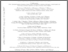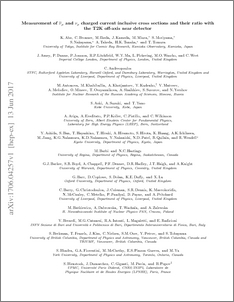# Measurement of $\barν_μ$ and $ν_μ$ charged current inclusive cross sections and their ratio with the T2K off-axis near detector

, T2K Collaboration (2017) Measurement of $\barν_μ$ and $ν_μ$ charged current inclusive cross sections and their ratio with the T2K off-axis near detector. Physical Review D, 96 (5). ISSN 1550-7998Preview
PDF (1706 (1).04257v1)
1706_1_.04257v1.pdf - Accepted Version

## Abstract

We report a measurement of cross section $\sigma(\nu_{\mu}+{\rm nucleus}\rightarrow\mu^{-}+X)$ and the first measurements of the cross section $\sigma(\bar{\nu}_{\mu}+{\rm nucleus}\rightarrow\mu^{+}+X)$ and their ratio $R(\frac{\sigma(\bar \nu)}{\sigma(\nu)})$ at (anti-)neutrino energies below 1.5 GeV. We determine the single momentum bin cross section measurements, averaged over the T2K $\bar{\nu}/\nu$-flux, for the detector target material (mainly Carbon, Oxygen, Hydrogen and Copper) with phase space restricted laboratory frame kinematics of $\theta_{\mu}$500 MeV/c. The results are $\sigma(\bar{\nu})=\left( 0.900\pm0.029{\rm (stat.)}\pm0.088{\rm (syst.)}\right)\times10^{-39}$ and $\sigma(\nu)=\left( 2.41\ \pm0.022{\rm{(stat.)}}\pm0.231{\rm (syst.)}\ \right)\times10^{-39}$ in units of cm$^{2}$/nucleon and $R\left(\frac{\sigma(\bar{\nu})}{\sigma(\nu)}\right)= 0.373\pm0.012{\rm (stat.)}\pm0.015{\rm (syst.)}$.

Item Type:
Journal Article
Journal or Publication Title:
Physical Review D
Subjects:
Departments:
ID Code:
86993
Deposited By:
Deposited On:
11 Jul 2017 09:00
Refereed?:
Yes
Published?:
Published